### Ohms Law Class 10th Science Lab Manual CBSE Solution

##### Question 1.Define a circuit diagram?Answer:The circuit diagram is a schematic representation which shows the arrangement of different devices or components by using their electrical symbol is called a circuit diagram.The basic circuit diagram is given as: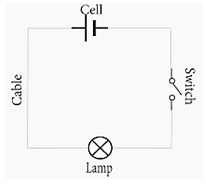Question 2.Define electric current. Give its SI unit?Answer:Electric current: It is the amount of charge following through a particular area in a unit time is called an electric current.The SI unit of current is 1A.Question 3.Define 1 A of current?Answer:The 1A of current is the flow of 1 coulomb of charge per second.Question 4.What is mA and μA?Answer:Ampere is the standard unit of current. The small units of current are:1. Milli-Ampere: 1 mA = 10-3A2. Micro-Ampere: 1Μa = 10-6 AQuestion 5.What is the direction of current in a circuit?Answer:The conventional direction of current is taken from positive terminal of the battery through the various circuit components & then to negative terminal.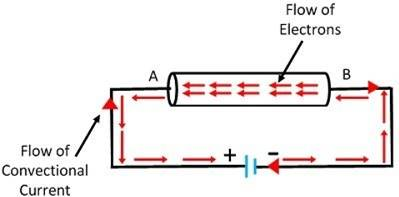Question 6.What are the functions of the following components of electric circuit?i. Ammeterii. Voltmeteriii. Resistoriv. Switchv. BatteryAnswer:The functions of the components are given as:i. Ammeter: It is a measuring instrument used to measure the current in a circuit.ii. Voltmeter: A voltmeter is an instrument used for measuring electrical potential difference between two points in an electric circuitiii. Resistor: It is an electrical component that limits or regulates the flow of electrical current in an electronic circuit.iv. Switch: A device for making and breaking the connection in an electric circuit.v. Battery: A battery is an electrochemical cell that can be charged electrically to provide a static potential for power.Question 7.What is the difference between electric potential & electric potential difference?Answer:i. The work done in bringing a unit positive charge from infinity to a point in an electric field is called as Electric potential of a point.ii. The potential difference between two points in an electric field or across the ends of a conductor is equal to the work done in bringing a charge from one point to anotherQuestion 8.What is resistance and factors affecting the resistance of a body?Answer:Resistance: It is the characteristic property of a conducting wire which resists the flow of electric current through it. Its SI unit is ohms.The factor affecting the resistance of a body are:1. Length of the wire.2. Cross-sectional area.3. The material of the conductor.4. The temperature of the conductor.Question 9.What is the difference between voltmeter & ammeter?Answer:Voltmeter is a very high resistance device which is used to measure the potential difference between two points. It is connected in parallel combination with the resistance across which the voltage drop has to be found.It is a very low resistance device which is used to measure the strength of the current in a circuit, it is always connected in series in a circuit.Question 10.How are ammeter and voltmeter connected in a circuit?Answer:A voltmeter is connected in parallel with a device to measure its voltage, while an ammeter is connected in series with a device to measure its current. The diagram is given below: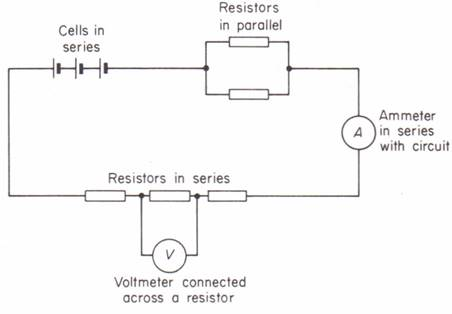Question 11.What is the ideal resistance of ammeter and voltmeter?Answer:The ideal resistance of voltmeter is infinite ohms.The ideal resistance of ammeter is 0 ohms.Question 12.What is Rheostat?Answer:it is a device which is used as a current controller in the circuit by changing its resistance. The Rheostat consists of wounds of nichrome wire in which each wound has a specific area and are connected in series with each other as we slide over this wounded wire the resistance of the rheostat changes.A picture of rheostat is attached here: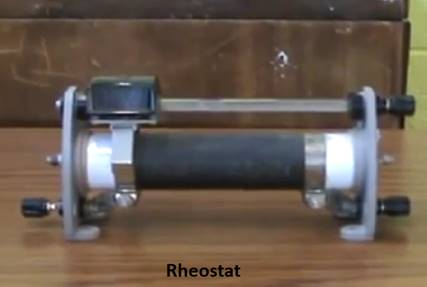Question 13.State Ohms law?Answer:If the physical conditions such as temperature, pressure remain the same, the current flowing through a conductor is directly proportional to the potential difference applied across it.Mathematically,V = IRWhere V is the potential differenceI is the currentR is the resistanceQuestion 14.Why it is never advised to keep the current flow for a long time in a resistance wire?Answer:The flowing current in the circuit led to the heating effect of current. As per joule law H = I2RT, the wire will be heated up which increases the resistance of wire.Question 15.What is an essential condition for current flow through the conductor?Answer:The necessary condition are:1. The circuit must be closed.2. There must be a source of electrical energy.Question 16.Why does current not flow in the circuit when we take out the plug from the key?Answer:Where there is no connectivity in the medium, the current does not flow. The air present in the gap caused due to removal of plug from the key is the bad conductor of electricity. Hence, the current does no flow.Question 17.What is battery eliminator?Answer:It is used in place of cell/ battery. With the help of step down transformer higher voltage of an alternating current is converted into a low voltage and then converted into direct current with the help of a rectifier.You might find battery eliminator in your school lab, it looks like this: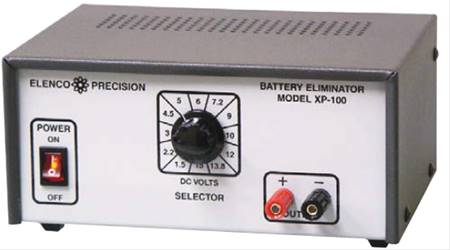Question 18.How do you mean by slope of a graph? What is the formula for slope?Answer:The slope is a measure of the steepness of any line connecting two points.For a line connecting any two points say P(x1, y1) and Q (x2, y2), the slope is given as :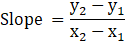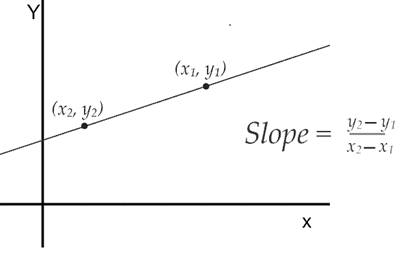Question 19.Which has more slope, A or B?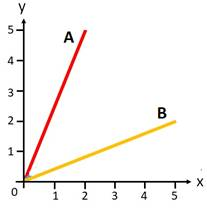Answer:More steep graph has more slope. Thus, A has more slope than B.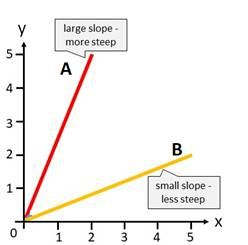Question 20.What is the resistance of a ideal ammeter?Answer:The resistance of an ideal ammeter is zero.

PDF FILE TO YOUR EMAIL IMMEDIATELY PURCHASE NOTES & PAPER SOLUTION. @ Rs. 50/- each (GST extra)

HINDI ENTIRE PAPER SOLUTION

MARATHI PAPER SOLUTION

SSC MATHS I PAPER SOLUTION

SSC MATHS II PAPER SOLUTION

SSC SCIENCE I PAPER SOLUTION

SSC SCIENCE II PAPER SOLUTION

SSC ENGLISH PAPER SOLUTION

SSC & HSC ENGLISH WRITING SKILL

HSC ACCOUNTS NOTES

HSC OCM NOTES

HSC ECONOMICS NOTES

HSC SECRETARIAL PRACTICE NOTES

# 2019 Board Paper Solution

HSC ENGLISH SET A 2019 21st February, 2019

HSC ENGLISH SET B 2019 21st February, 2019

HSC ENGLISH SET C 2019 21st February, 2019

HSC ENGLISH SET D 2019 21st February, 2019

SECRETARIAL PRACTICE (S.P) 2019 25th February, 2019

HSC XII PHYSICS 2019 25th February, 2019

CHEMISTRY XII HSC SOLUTION 27th, February, 2019

OCM PAPER SOLUTION 2019 27th, February, 2019

HSC MATHS PAPER SOLUTION COMMERCE, 2nd March, 2019

HSC MATHS PAPER SOLUTION SCIENCE 2nd, March, 2019

SSC ENGLISH STD 10 5TH MARCH, 2019.

HSC XII ACCOUNTS 2019 6th March, 2019

HSC XII BIOLOGY 2019 6TH March, 2019

HSC XII ECONOMICS 9Th March 2019

SSC Maths I March 2019 Solution 10th Standard11th, March, 2019

SSC MATHS II MARCH 2019 SOLUTION 10TH STD.13th March, 2019

SSC SCIENCE I MARCH 2019 SOLUTION 10TH STD. 15th March, 2019.

SSC SCIENCE II MARCH 2019 SOLUTION 10TH STD. 18th March, 2019.

SSC SOCIAL SCIENCE I MARCH 2019 SOLUTION20th March, 2019

SSC SOCIAL SCIENCE II MARCH 2019 SOLUTION, 22nd March, 2019

XII CBSE - BOARD - MARCH - 2019 ENGLISH - QP + SOLUTIONS, 2nd March, 2019

# HSCMaharashtraBoardPapers2020

(Std 12th English Medium)

HSC ECONOMICS MARCH 2020

HSC OCM MARCH 2020

HSC ACCOUNTS MARCH 2020

HSC S.P. MARCH 2020

HSC ENGLISH MARCH 2020

HSC HINDI MARCH 2020

HSC MARATHI MARCH 2020

HSC MATHS MARCH 2020

# SSCMaharashtraBoardPapers2020

(Std 10th English Medium)

English MARCH 2020

HindI MARCH 2020

Hindi (Composite) MARCH 2020

Marathi MARCH 2020

Mathematics (Paper 1) MARCH 2020

Mathematics (Paper 2) MARCH 2020

Sanskrit MARCH 2020

Sanskrit (Composite) MARCH 2020

Science (Paper 1) MARCH 2020

Science (Paper 2)

Geography Model Set 1 2020-2021

MUST REMEMBER THINGS on the day of Exam

Are you prepared? for English Grammar in Board Exam.

Paper Presentation In Board Exam

How to Score Good Marks in SSC Board Exams

Tips To Score More Than 90% Marks In 12th Board Exam

How to write English exams?

How to prepare for board exam when less time is left

How to memorise what you learn for board exam

No. 1 Simple Hack, you can try out, in preparing for Board Exam

How to Study for CBSE Class 10 Board Exams Subject Wise Tips?

JEE Main 2020 Registration Process – Exam Pattern & Important Dates

NEET UG 2020 Registration Process Exam Pattern & Important Dates

How can One Prepare for two Competitive Exams at the same time?

8 Proven Tips to Handle Anxiety before Exams!